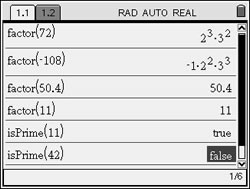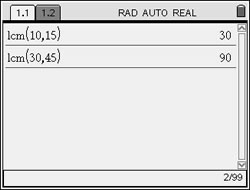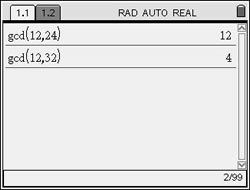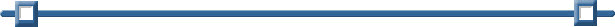Prime Factors, LCM and GCF

Finding Prime Factors:

 factor(rationalNumber) returns the rational number factored into primes. If you are simply trying to decide if a number is prime, use isPrime( ). Example:   Find the prime factors of 72. From a Calculator Page: MENU - #2 Number - #2 FactorNOTE: According to the manual, the computing time for composite numbers grows exponentially with the number of digits in the second-largest factor. Factoring a 30-digit integer could take more than a day and a 100-digit number could take more than a century.

Finding the Least Common Multiple (LCM):

 lcm(Number1, Number2) will return the least common multiple of the two numeric values. Only accepts two values at a time. Example:   Find the LCM for 10, 15, and 45. From a Calculator Page: MENU - #2 Number #3 Least Common Multiple   Since LCM can only accept two entries at a time, find the LCM of the first two values, and then find the LCM of your answer and the third value.Finding the Greatest Common Factor (GCF):

 gcd(Number1, Number2) will return the greatest common divisor of the two numeric values. Only accepts two values at a time. NOTE:  The calculator refers to the GCF as the GCD, greatest common divisor. Example:   Find the GCF (or GCD) for 12, 24, and 32. From a Calculator Page: MENU - #2 Number #4 Greatest Common Divisor  Since GCD can only accept two entries at a time, find the GCD of the first two values, and then find the GCD of your answer and the third value.